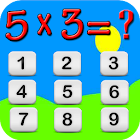Multiplication Game

• SEARCH TYPE

All Android applications categories

All Android games categoriesMultiplication Game

by: 2 10

10 Users
rating

Screenshots

Description

This app contains multiplication of digits and alphabetic which is easy to learn and identity of integers will help you to learn quickly.
This game is strongly recommended for you to learn multiplication and learn English.
multiplication game with excellent attractive graphics.
This game also contains learning videos.
You will learn many things with this game.
Math multiplication game is very easy to use with rich graphics
Math multiplication with sounds and timer game.
You will learn how to count integers, how to sum integers.
This is very basic mathematics for you to learning English and mathematics.
You will learn to play sum game with English learning.

Math multiplication Game Features:
- Kids learning game
- math multiplication for every age person
- Math learning game free
- Learn 2 count
- Kids learning game
- math learning game
- Basic counting game
- kids multiply game
- Alphabetic learning game
- English math learning
- Basic math learning game free
- Math and English basic knowledge
- Birds counting in English
- Cartoon videos
- Learn to multiplication game
- Basic mathematics
- Kids math
- Basic Kids multiplication game
- Math Game with scores
- Learn to count
- Easy math for Kids
- Counting videos
- Cartoon learning app
- Alphabetic Learning
- Math and English learning
- multiply game
- Best Learning Videos Added
- Alphabets Learning Added
- Numbers and Alphabets Added
- Basic Math multiplication game
- Learning Videos
- multiplication tables game
- Improved Design
- Hindi Poems
- Kids learning app
- Best math sum learning app
- Math game with scoreboard
- easy multiplication tables
- Basic knowledge of math
- Best multiply game with timer
- Learn with cartoons
- Basic knowledge about math and English
- Kids maths multiplication
- English Poems
- Urdu poems
- poem videos
- Kids poems
- Learning poems
- Alphabetic videos
- multiply game
- multiplication tables game
- Mathematics for all
- Easy math for learners
- Bug fixed
- Kids learning videos
- Math learning game
- Learn 2 multiplication
- Math with pictures
- Math words counting
- Math & English learning game
- Learn with pictures
- English and Math games
- Education with cartoons